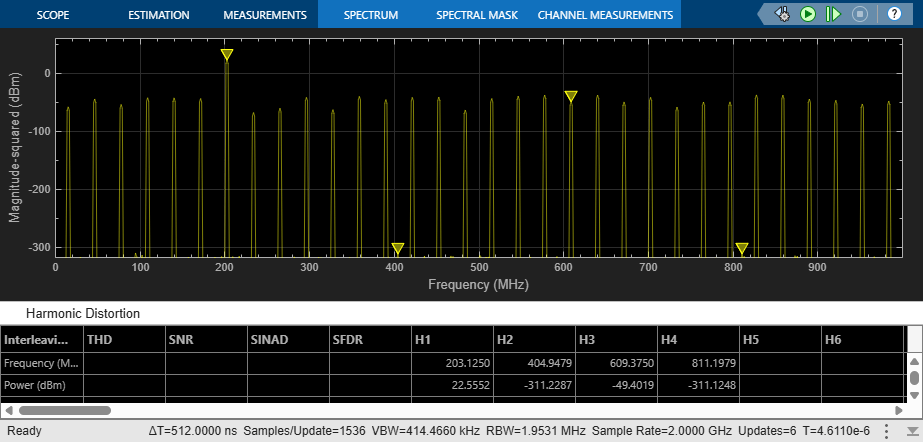# Design and Evaluate Interleaved ADC

This interleaved ADC model highlights some of the typical impairments introduced by data converters and their effects on a larger system.

### Model

In this example, interleave two simple ADCs based on the model Analyzing Simple ADC with Impairments to create the equivalent of one ADC operating at 2X the individual ADC sampling rate. Use a two-tone test signal at `200` MHz and `220` MHz as the input to verify the distortion introduced by the ADC operation.

```model = 'interleaved_adc'; open_system(model) set_param([model '/ADC_1 at 1G SPS'],'jitter','off'); set_param([model '/ADC_1 at 1G SPS'],'nonlinearity','off'); set_param([model '/ADC_1 at 1G SPS'],'quantization','off'); set_param([model '/ADC_2 at 1G SPS'],'jitter','off'); set_param([model '/ADC_2 at 1G SPS'],'nonlinearity','off'); set_param([model '/ADC_2 at 1G SPS'],'quantization','off'); set_param([model '/Offset Delay'],'DelayTime','.5/Fs_adc'); set_param([model '/Two Tone Sine Wave'],'Amplitude','.5'); set_param([model, '/Input Switch'], 'sw', '1'); sim(model) ```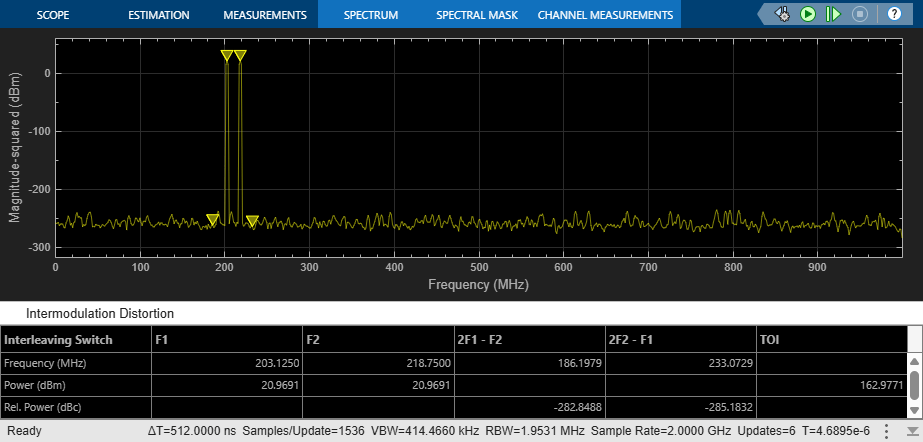To bypass the impairments, use appropriate switch positions inside the ADC blocks. The ADC behavior is purely ideal. The two ADCs in the top-level model are identical with the exception that the noise generators in each ADC have different seeds to make the noise uncorrelated.

Each ADC operates at `1` GHz rate, set by the MATLAB® variable Fs_adc defined in the initialization callback of this model. The operating rate of the ADCs is indicated by the green signals and blocks in the diagram. The input signal of the second ADC is delayed by an amount equal to half a period of the ADC sampling frequency.

### Timing Imperfection

The precision of the timing between the individual ADCs is critical. To see the effect of a timing mismatch, open the Offset Delay block and simply add `10` ps to the delay value.

```set_param([model '/Offset Delay'],'DelayTime','.5/Fs_adc + 10e-12'); ```

The `10` ps error causes a significant degradation of the ADC performance, even though both ADCs are perfectly ideal. To compensate for the performance degradation, some form of drift compensation is necessary. For more information, see Time-Interleaved ADC Error Correction.

```sim(model) ```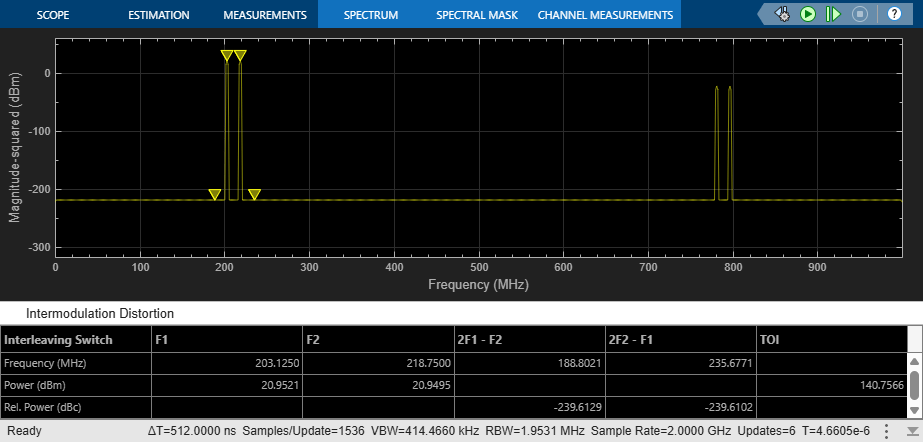### Effect of Aperture Jitter

Remove the fixed offset of `10` ps and enable the aperture jitter impairment in each of the ADC subsystems.

```set_param([model '/Offset Delay'],'DelayTime','.5/Fs_adc'); set_param([model '/ADC_1 at 1G SPS'],'jitter','on'); set_param([model '/ADC_2 at 1G SPS'],'jitter','on'); ```

The noise around the two-tone test signal at `200` MHz is expected, as a direct result of the ADC jitter. The additional noise around `800` MHz is the result of interleaving two uncorrelated noise sources.

```sim(model) ```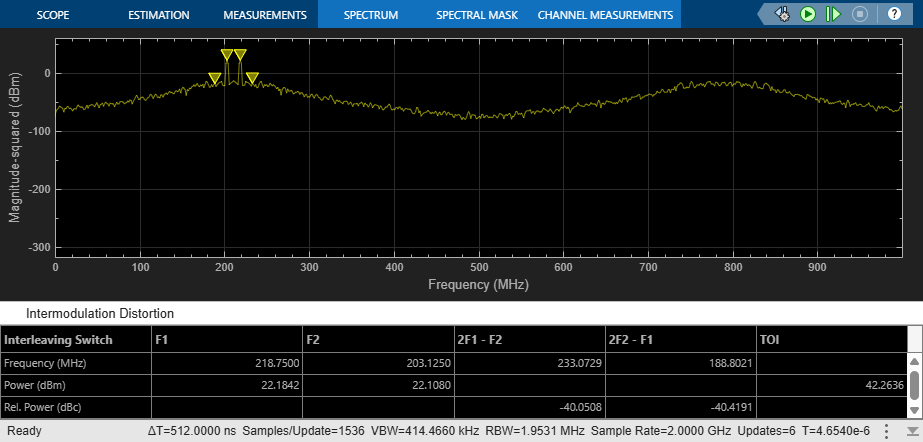### Effect of Nonlinearity

Remove the jitter impairment and activate the nonlinearity impairment in both ADCs.

```set_param([model '/ADC_1 at 1G SPS'],'jitter','off'); set_param([model '/ADC_2 at 1G SPS'],'jitter','off'); set_param([model '/ADC_1 at 1G SPS'],'nonlinearity','on'); set_param([model '/ADC_2 at 1G SPS'],'nonlinearity','on'); ```

The spectrum now shows 3rd order IMD products around two tones and harmonically related spurs around the `600` MHz region.

```sim(model) ```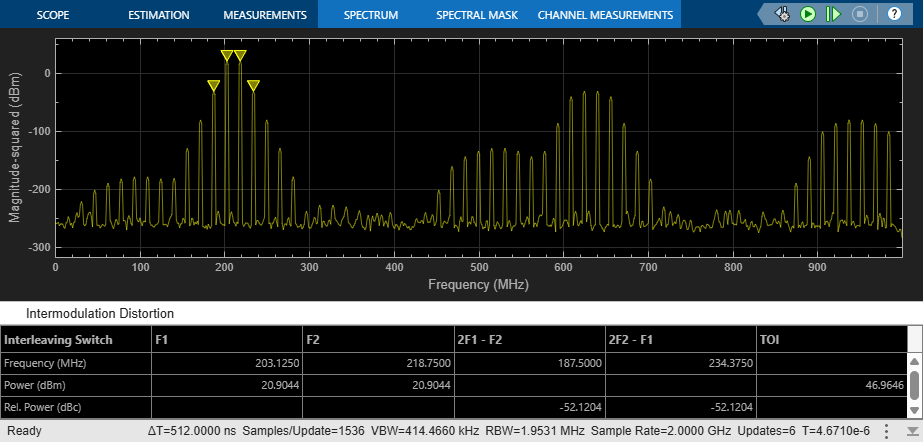Even though the ADC nonlinear effects are identical and create exactly the same odd order components, there is actually some cancellation of terms. If just one nonlinearity is enabled, the resulting spectrum is worse than when both ADCs are nonlinear.

```set_param([model '/ADC_2 at 1G SPS'],'nonlinearity','off'); sim(model) ```### Effect of Quantization and Saturation

Remove the linearity impairment and activate the quantization. The quantizer is set to `9` bits, and the signal level is close to the full scale of `+/-1`, which can be seen in the input Time Scope.

```set_param([model '/ADC_1 at 1G SPS'],'nonlinearity','off'); set_param([model '/ADC_2 at 1G SPS'],'nonlinearity','off'); set_param([model '/ADC_1 at 1G SPS'],'quantization','on'); set_param([model '/ADC_2 at 1G SPS'],'quantization','on'); ```

The spectrum shows the noise floor increasing as an effect of quantization.

```sim(model) ```Multiply the two tone test signal by a factor of 1.2. The increased amplitude saturates each ADC, producing a clipped waveform and a dirty spectrum.

```set_param([model '/Two Tone Sine Wave'],'Amplitude','.5*1.2'); sim(model) ```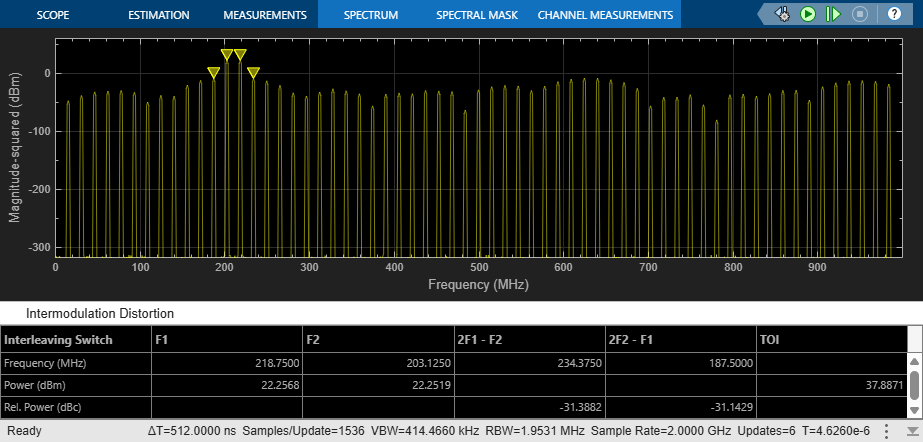### ENOB, SFDR, and Other Single Tone Measurements

ADCs are often characterized by their Effective Number of Bits (ENoB), Spurrious-Free Dynamic Range (SFDR), and other similar measurements.

These quantities are derived from a single tone test. To change the ADC's input from the Two Tone Sine Wave source to the Single Tone Sine Wave source and back, double click on the Input Switch. This test uses a single sine wave with a frequency of `200` MHz.

```set_param([model, '/Input Switch'], 'sw', '0'); ```

The ADC AC Measurement block from the Mixed-Signal Blockset™ measures conversion delay, SINAD (the ratio of signal to noise and distortion), SFDR, SNR (Signal to Noise Ratio), ENOB and the ADC's output noise floor.

```set_param([model, '/ADC AC Measurement'], 'Commented', 'off'); ```

This block requires a rising edge on its start and ready ports for every conversion that the ADC makes. In this model, these are provided by a `4` GHz pulse generator. To use the ADC AC Measurement block in this model, uncomment the block by right clicking on it and selecting "Uncomment" from the menu. The expected ENOB from a dynamic range of `2` and a least significant bit value (quantization interval) of `2^-8` is `9` bits.

```sim(model); disp(interleaved_adc_output) ```
``` SNR: 316.4415 SFDR: 58.7451 SINAD: 58.7451 ENOB: 9.4660 NoiseFloor: -351.8406 MaxDelay: 0 MeanDelay: 0 MinDelay: 0 ```